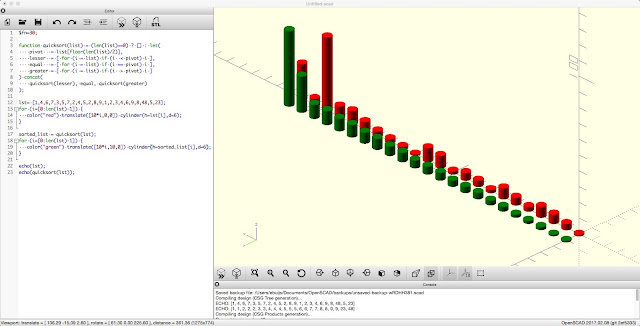## Lists

This is the second part in a series on OpenSCAD (here is a link to the first). This series will not cover the very basics of OpenSCAD, there is plenty of good material on that, but it's for those who want to advance beyond these basics. This part is about Lists, sequences of OpenSCAD values. These are very important in OpenSCAD because they are the equivalent of arrays. They're also referred to as vectors. Lists can contain values numbers, booleans and strings. For most examples below version 2015.03 is required.Quicksort example from the OpenSCAD User Manual demonstrates how to manipulate a list. The red row of cylinders represents the unsorted list while the green row represents the sorted list.

First let's create some lists by typing the following in the OpenSCAD editor.

lst = [1,2,3,4,5,6,7,8,9]; //a list of numbers
lst2 = ["red","yellow","blue"]; //a list of strings
lst3 = [false,true,undef]; //a list of booleans
echo(lst);
echo(lst2);
echo(lst3);

In OpenSCAD the user is able to automatically generate lists with for, if and let. A couple of examples

lst4 = [for (i = [0:9]) i*i];

This generates a list of [0, 1, 4, 9, 16, 25, 36, 49, 64, 81], so for every i the results is i multiplied by i. OpenSCAD even allows nested loops

lst5 = [for (i = [0:2]) for (j =[0:2]) for (n=[0:2]) [i,j,n]];

This generates a list of a long list of vectors ECHO: [[0, 0, 0], [0, 0, 1], [0, 0, 2], [0, 1, 0], [0, 1, 1], [0, 1, 2], [0, 2, 0], [0, 2, 1], [0, 2, 2], [1, 0, 0], [1, 0, 1], [1, 0, 2], [1, 1, 0], [1, 1, 1], [1, 1, 2], [1, 2, 0], [1, 2, 1], [1, 2, 2], [2, 0, 0], [2, 0, 1], [2, 0, 2], [2, 1, 0], [2, 1, 1], [2, 1, 2], [2, 2, 0], [2, 2, 1], [2, 2, 2]]

## Manipulate a list

A list in OpenSCAD can be manipulated with a function. Manipulation is needed in order to make changes to the list. Since OpenSCAD is a Functional Language we can't just for example change value in the list. We need a function to do this. Lets assume we want to create a partial list from an existing list. We then create the following function:

function partial(list,start,end) = [for (i = [start:end]) list[i]];
echo(partial(lst,1,8));

In the Console of OpenSCAD the following is displayed ECHO: [2, 3, 9, 5, 6, 7, 8, 4]. The function has three parameters: a list, a start number and an end number. The function iterates through the list and then creates a new list with the items with indices 1 to 8. Note that the first item in the list with index 0 has been excluded from the new list. Another example.

echo(partial(lst,4,7));

This prompts ECHO: [5,6,7,8], a list with the 4th to the 7th item of lst. Remember that lst itself hasn't changed (you can check this yourself). Next we write a function to remove a number in a list.

function remove_item(list,position) = [for (i = [0:len(list)-1]) if (i != position) list[i]];
echo(remove_value(lst,2));

This prompt ECHO: [1,2,4,5,6,7,8,9], the third item in the list has been removed. The function, that has the parameters list and position, loops through the items of the list and if the index i is not equal to the parameter position it is added to the new list. In this example it means that the item with the index 2 is not part of the new list. The following function inserts a value instead of removing it.

function insert(list,value,position) = let (l1 = partial(list,0,position-1), l2 = partial(list,position,len(list)-1)) concat(add_value(l1,value),l2);
echo(insert(lst,10,4));

It cuts the list on the position 4 in two new lists, inserts a number of 10 between the two lists, using the function partial that was created above. The result in the console is: ECHO: [1, 2, 3, 4, 10, 5, 6, 7, 8, 9]. BTW: It's entirely possible that there's an easier way to do this.

The last and most complicated example is taken from the OpenSCAD User Manual and implements the quicksort algorithm. The quicksort function uses an unsorted list as input and produces a sorted list with a recursive method.

lst6 = [1,4,6,7,3,5,7,2]; //a list of numbers
function quicksort(list) = !(len(list)>0) ? [] : let(
pivot   = list[floor(len(list)/2)],
lesser  = [ for (i = list) if (i  < pivot) i ],
equal   = [ for (i = list) if (i == pivot) i ],
greater = [ for (i = list) if (i  > pivot) i ]
) concat(
quicksort(lesser), equal, quicksort(greater)
);

echo(lst6);
echo(quicksort(lst6));

It produces the following output ECHO: [1,2,3,4,5,6,7,7]. The function starts with a base case !(len(list)>0) ? [] meaning if a the length of list is 0 return an empty list (or the boolean value false) else continue. Next a pivot is determined being the number in the middle of the list, in this case the number 3. Next all numbers in the list smaller than 3 are placed in a list lesser, all numbers equal to 3 are placed in a list equal and all numbers larger than 3 are placed in a list greater. These three list are then concatenated to a new list however, and now we get to the recursive parts, the lesser and greater lists are the new parameters for quicksort. This iteration continues until the base case, the length of the list is equal to zero or less, is reached.

Understanding lists and list manipulation is essential to advance in OpenSCAD. Without it would for instance be near impossible to work with the all important vectors in both 2D and 3D. But also in other areas, such as iterating through a row, lists are essential.